# Numerical and Algebraic Expressions

Students venture into the Expressions and Equations domain, using variables to represent unknown or changing quantities, and using properties of operations to investigate equivalent expressions.

Math

Unit 5

## Unit Summary

In Unit 5, 6th grade students venture into the Expressions and Equations domain for the first time, extending on their understanding of arithmetic to see how it applies to algebraic expressions. They start with numerical expressions with exponents, rewriting the expressions into simpler forms until a final value is determined. Students then use variables in expressions to represent quantities that are unknown or quantities that change. Using the properties of operations, students will investigate what makes expressions equivalent to others, a concept that is threaded throughout the middle school Expressions and Equations domain. Throughout this unit, students pay close attention to the structure of expressions, understanding the role of parentheses, the order of operations, and the way expressions are described verbally (MP.7). A note on fluency: Evaluating expressions provides a good opportunity for students to continue developing and to demonstrate fluency with decimal operations. Several problems throughout the unit include decimal values; include additional problems in practice for students as needed.

In elementary school, students used variables to represent unknown quantities, and they evaluated and described numerical expressions without exponents. They used the commutative property to enhance their understanding of multiplication and addition, and they used the distributive property when modeling partial areas. All of these concepts come together and support student understanding in this unit.

Immediately following this unit, students will start a unit on Equations and Inequalities, where they will use algebra to model and solve real-world problems. They will also revisit percentages using new skills with expressions and equations to efficiently solve percent problems. In 7th grade and 8th grade, students continue to simplify and solve more complex expressions and equations using the same tools learned in this unit.

Pacing: 16 instructional days (12 lessons, 3 flex days, 1 assessment day)

Fishtank Plus for Math

Unlock features to optimize your prep time, plan engaging lessons, and monitor student progress.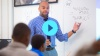## Assessment

The following assessments accompany Unit 5.

### Pre-Unit

Have students complete the Pre-Unit Assessment and Pre-Unit Student Self-Assessment before starting the unit. Use the Pre-Unit Assessment Analysis Guide to identify gaps in foundational understanding and map out a plan for learning acceleration throughout the unit.

### Mid-Unit

Have students complete the Mid-Unit Assessment.

### Post-Unit

Use the resources below to assess student understanding of the unit content and action plan for future units.

Expanded Assessment Package

Use student data to drive your planning with an expanded suite of unit assessments to help gauge students’ facility with foundational skills and concepts, as well as their progress with unit content.

## Unit Prep

### Intellectual Prep

Unit Launch

Prepare to teach this unit by immersing yourself in the standards, big ideas, and connections to prior and future content. Unit Launches include a series of short videos, targeted readings, and opportunities for action planning.#### Internalization of Standards via the Post-Unit Assessment

• Take the Post-Unit Assessment. Annotate for:
• Standards that each question aligns to
• Strategies and representations used in daily lessons
• Relationship to Essential Understandings of unit
• Lesson(s) that Assessment points to

#### Internalization of Trajectory of Unit

• Read and annotate the Unit Summary.
• Notice the progression of concepts through the unit using the Lesson Map.
• Essential Understandings
• Connection to Post-Unit Assessment questions
• Identify key opportunities to engage students in academic discourse. Read through our Teacher Tool on Academic Discourse and refer back to it throughout the unit.

#### Unit-Specific Intellectual Prep

 Model Example Tape diagram $$4-m$$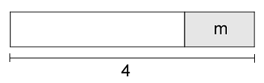$$m-4$$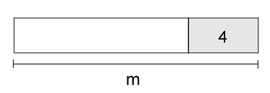$$3x+2=x+x+x+2$$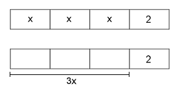Area model $$2\times5^2$$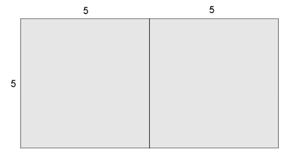$$2+5^2$$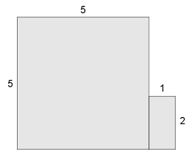### Essential Understandings

• Numerical expressions can be evaluated using the order of operations. Paying close attention to the structure of an expression, such as what role the parentheses play or where the exponents are, can provide insight into how an expression is evaluated to a single number.
• When two algebraic expressions are equivalent, they are equal for any value of the variable(s).
• Equivalent expressions can be identified and generated by using properties of operations, specifically the distributive property and the commutative property.
• Algebraic expressions can be used to represent real-world situations where variables represent unknown quantities or quantities that vary.

### Vocabulary

algebraic expression

commutative property

distributive property

equivalent

numerical expression

order of operations

term

variable

To see all the vocabulary for Unit 5, view our 6th Grade Vocabulary Glossary.

## Lesson Map

Topic A: Numerical Expressions with Exponents

Topic B: Introduction to Algebraic Expressions

Topic C: Equivalent Expressions & Applications

## Common Core Standards

Key

Major Cluster

Supporting Cluster

### Core Standards

#### Expressions and Equations

• 6.EE.A.1 — Write and evaluate numerical expressions involving whole-number exponents.
• 6.EE.A.2 — Write, read, and evaluate expressions in which letters stand for numbers.
• 6.EE.A.2.A — Write expressions that record operations with numbers and with letters standing for numbers. For example, express the calculation "Subtract y from 5" as 5 - y.
• 6.EE.A.2.B — Identify parts of an expression using mathematical terms (sum, term, product, factor, quotient, coefficient); view one or more parts of an expression as a single entity. For example, describe the expression 2 (8 + 7) as a product of two factors; view (8 + 7) as both a single entity and a sum of two terms.
• 6.EE.A.2.C — Evaluate expressions at specific values of their variables. Include expressions that arise from formulas used in real-world problems. Perform arithmetic operations, including those involving whole-number exponents, in the conventional order when there are no parentheses to specify a particular order (Order of Operations). For example, use the formulas V = s³ and A = 6 s² to find the volume and surface area of a cube with sides of length s = 1/2.
• 6.EE.A.3 — Apply the properties of operations to generate equivalent expressions. For example, apply the distributive property to the expression 3 (2 + x) to produce the equivalent expression 6 + 3x; apply the distributive property to the expression 24x + 18y to produce the equivalent expression 6 (4x + 3y); apply properties of operations to y + y + y to produce the equivalent expression 3y.
• 6.EE.A.4 — Identify when two expressions are equivalent (i.e., when the two expressions name the same number regardless of which value is substituted into them). For example, the expressions y + y + y and 3y are equivalent because they name the same number regardless of which number y stands for.
• 6.EE.B.6 — Use variables to represent numbers and write expressions when solving a real-world or mathematical problem; understand that a variable can represent an unknown number, or, depending on the purpose at hand, any number in a specified set.

• 5.MD.C.5.B

• 4.NBT.B.5

• 4.OA.A.2
• 4.OA.A.3
• 5.OA.A.1
• 5.OA.A.2

• 6.EE.B.5
• 6.EE.B.7
• 6.EE.B.8
• 6.EE.C.9
• 7.EE.A.1
• 7.EE.B.4

### Standards for Mathematical Practice

• CCSS.MATH.PRACTICE.MP1 — Make sense of problems and persevere in solving them.

• CCSS.MATH.PRACTICE.MP2 — Reason abstractly and quantitatively.

• CCSS.MATH.PRACTICE.MP3 — Construct viable arguments and critique the reasoning of others.

• CCSS.MATH.PRACTICE.MP4 — Model with mathematics.

• CCSS.MATH.PRACTICE.MP5 — Use appropriate tools strategically.

• CCSS.MATH.PRACTICE.MP6 — Attend to precision.

• CCSS.MATH.PRACTICE.MP7 — Look for and make use of structure.

• CCSS.MATH.PRACTICE.MP8 — Look for and express regularity in repeated reasoning.

Unit 4

Rational Numbers

Unit 6

Equations and Inequalities

## Request a Demo

See all of the features of Fishtank in action and begin the conversation about adoption.

Yes

No